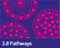# maths300 lessons

Gladstone Cluster teachers accessed maths300 lessons from the Education Services Australia website as the investigative approach in many lessons suited the Cluster focus.

The maths300 lessons referred to in this Make It Count resource by the Cluster are listed below with links to stories by the relevant teachers about the lessons.

1. Radioactivity (Lesson No. 7) Years 6-12  Chance and data, Number  This lesson addresses the hugely important current social issue of the development of Uranium mines and the problems of radioactive waste. Radioactive waste involves the concept of a half-life and exponential decay functions. All radioactive material is described in terms of its half-life. Arising from this community concern the students 'pretend' to be uranium atoms and model the decay process. A computer simulation then provides an investigative tool to explore the underlying concepts of 'half-life' and exponential decay. Students discover just how long some of this material can stay in the environment. The mathematical aspects of this lesson should be seen as supporting a joint study of the topic with other subject disciplines to bring out the full range issues.(maths300)
2. Estimation Walks (Lesson No: 13) Years 4-9  Measurement, Number  This lesson addresses the hugely important current social issue of the development of Uranium mines and the problems of radioactive waste. Radioactive waste involves the concept of a half-life and exponential decay functions. All radioactive material is described in terms of its half-life. Arising from this community concern the students 'pretend' to be uranium atoms and model the decay process. A computer simulation then provides an investigative tool to explore the underlying concepts of 'half-life' and exponential decay. Students discover just how long some of this material can stay in the environment. The mathematical aspects of this lesson should be seen as supporting a joint study of the topic with other subject disciplines to bring out the full range issues. (maths300)  See See Sandra's significant episode to find out how she went with the lesson.
3. Chocolate Cake (Lesson No. 135) Years 4-10  Measurement, Number, Space  In the context of a make believe party at which six rectangular chocolate cakes are shared, the students investigate making fractions. The cakes are represented by sheets of A4 paper and different groups of students are challenged to divide different numbers of cakes into various fractions. Measurement, folding, discussion and consensus are encouraged. The lesson is a wonderful introduction to a fraction unit because it reveals a considerable amount about the students' current understanding of the topic. (maths300)

The key outcome for me was the power of a meaningful and conceptually relevant context for investigating mathematical ideas. In this lesson the concept of radioactivity was not a contrived or artificial example to illustrate the mathematical concept, but it was a meaningful case where the mathematics was integral.

See Terry's significant episode to find out how he went with the lesson.